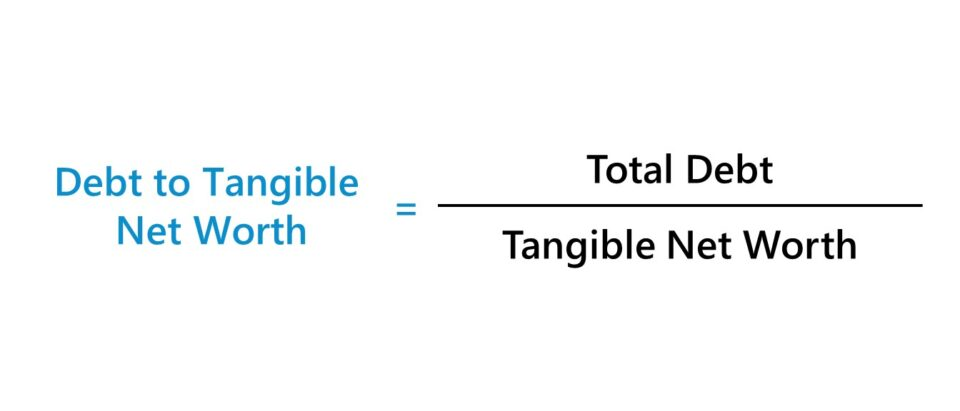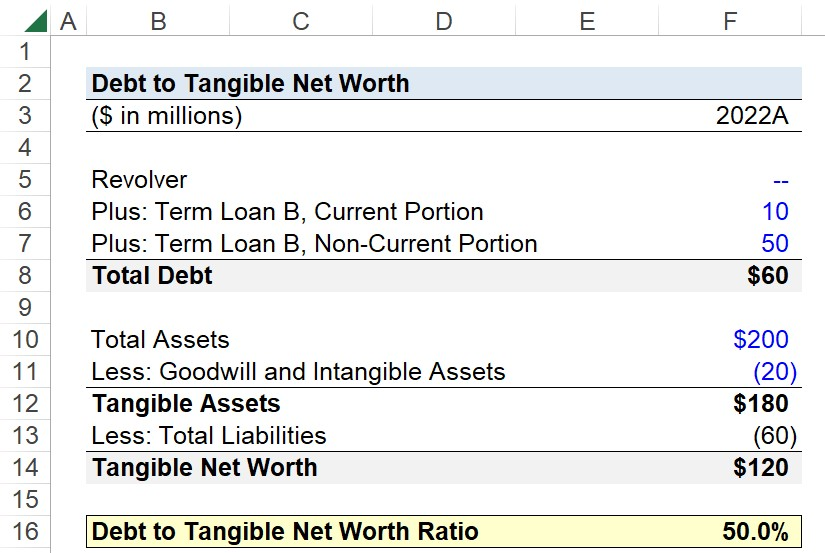Welcome to Wall Street Prep! Use code at checkout for 15% off.# Debt to Tangible Net Worth

Guide to Understanding Debt to Tangible Net Worth## How to Calculate Debt to Tangible Net Worth (Step-by-Step)

The debt to tangible net worth metric is the ratio between a company’s total outstanding debt balance and its tangible net worth.

• Debt Balance → The total debt outstanding of a company refers to the unmet debt obligations sitting on its balance sheet. Capital raising in the form of debt financing is a method for corporations to raise funding to finance day-to-day operations and the purchase of fixed assets, i.e. capital expenditures (Capex).
• Tangible Net Worth → The tangible net worth is the value of a company’s total assets according to its balance sheet minus any “non-physical” intangible assets, such as goodwill, intellectual property, patents, and more.

From the viewpoint of a management team amid liquidity risk management, tangible assets are viewed more favorably than intangible assets because tangible assets tend to be more marketable and more likely to possess some value post-liquidation, which would then be distributed to claim holders.

## Debt to Tangible Net Worth Formula

The formula for calculating the debt to tangible net worth is as follows:

Debt to Tangible Net Worth = Total Debt ÷ Tangible Net Worth

Where:

• Total Debt = Σ Debt Obligations and Interest-Bearing Securities
• Tangible Net Worth = (Total Assets – Intangible Assets) – Total Liabilities

## Debt to Tangible Net Worth vs. Debt to Equity Ratio (D/E)

The debt to tangible net worth ratio is regarded as a more conservative measure of a company’s financial state.

For instance, the debt to equity ratio (D/E) is one of the most common methods to evaluate the credit risk profile of a company. Unlike the debt to tangible net worth metric, however, the D/E ratio makes no adjustments to remove the value of intangible assets.

The D/E ratio measures the amount of debt financing utilized by a company to finance its asset base (i.e. resources) relative to the value of shareholders’ equity.

Generally, a responsible, risk-averse borrower should strive to maintain a ratio of total debt to tangible net worth of less than 1.0x (or 100%).

If a company’s debt to tangible net worth exceeds 1.0x, that would be viewed as a potential red flag and a cause for concern to lenders in terms of the perceived credit risk.

Since the total debt outstanding belonging to the company exceeds its tangible net worth, the risk of default increases substantially, i.e. the company may have a sub-optimal capital structure.

## Debt to Tangible Net Worth Calculator – Excel Template

We’ll now move to a modeling exercise, which you can access by filling out the form below.Submitting...

## Step 1. Total Debt Calculation Example

Suppose you’re tasked with calculating the debt to tangible net worth ratio of a company given the following operating assumptions for fiscal year 2022.

Financial Leverage Assumptions:

• Revolver = \$0 million
• Term Loan B, Current Portion = \$10 million
• Term Loan B, Non-Current Portion = \$50 million

The revolving credit facility was extended by a corporate bank, but the company has not needed to draw from the revolver. Hence, the balance of zero.

The next debt tranche is the term loan B, which is split into two categories based on the maturity date.

1. Current Portion: Maturity <12 Months
2. Non-Current Portion: Maturity >12 Months

The sum of the current and non-current portion of the term loan B is the total debt outstanding, which we’ll assume is the only liability on the company’s balance sheet, for the sake of simplicity.

• Total Debt = \$0 million + \$10 million + \$50 million = \$60 million

## Step 2. Tangible Net Worth Calculation

The calculation of our company’s tangible net worth starts with total assets, which we’ll assume is \$200 million.

Of the \$200 million in asset value, \$20 million is attributable to goodwill and other intangible assets.

Balance Sheet Assumptions:

• Total Assets = \$200 million
• Goodwill and Intangible Assets = \$20 million

Given those two inputs, the difference represents the value of our company’s tangible assets.

• Tangible Assets = \$200 million – \$20 million = \$180 million

From there, we’ll subtract total liabilities (i.e. the total debt balance) from the tangible assets balance, which results in a tangible net worth of \$120 million.

• Tangible Net Worth = \$180 million – \$60 million = \$120 million

In closing, we’ll divide our company’s total outstanding debt balance by its tangible net worth, which comes out to 50%.

• Debt to Tangible Net Worth = \$60 million ÷ \$120 million = 0.50, or 50.0%

The debt to tangible net worth ratio of 0.5x, or 50.0%, implies that approximately half of the company’s tangible net worth was funded using debt capital provided by lenders.Step-by-Step Online Course

### Everything You Need To Master Financial Modeling

Enroll in The Premium Package: Learn Financial Statement Modeling, DCF, M&A, LBO and Comps. The same training program used at top investment banks.